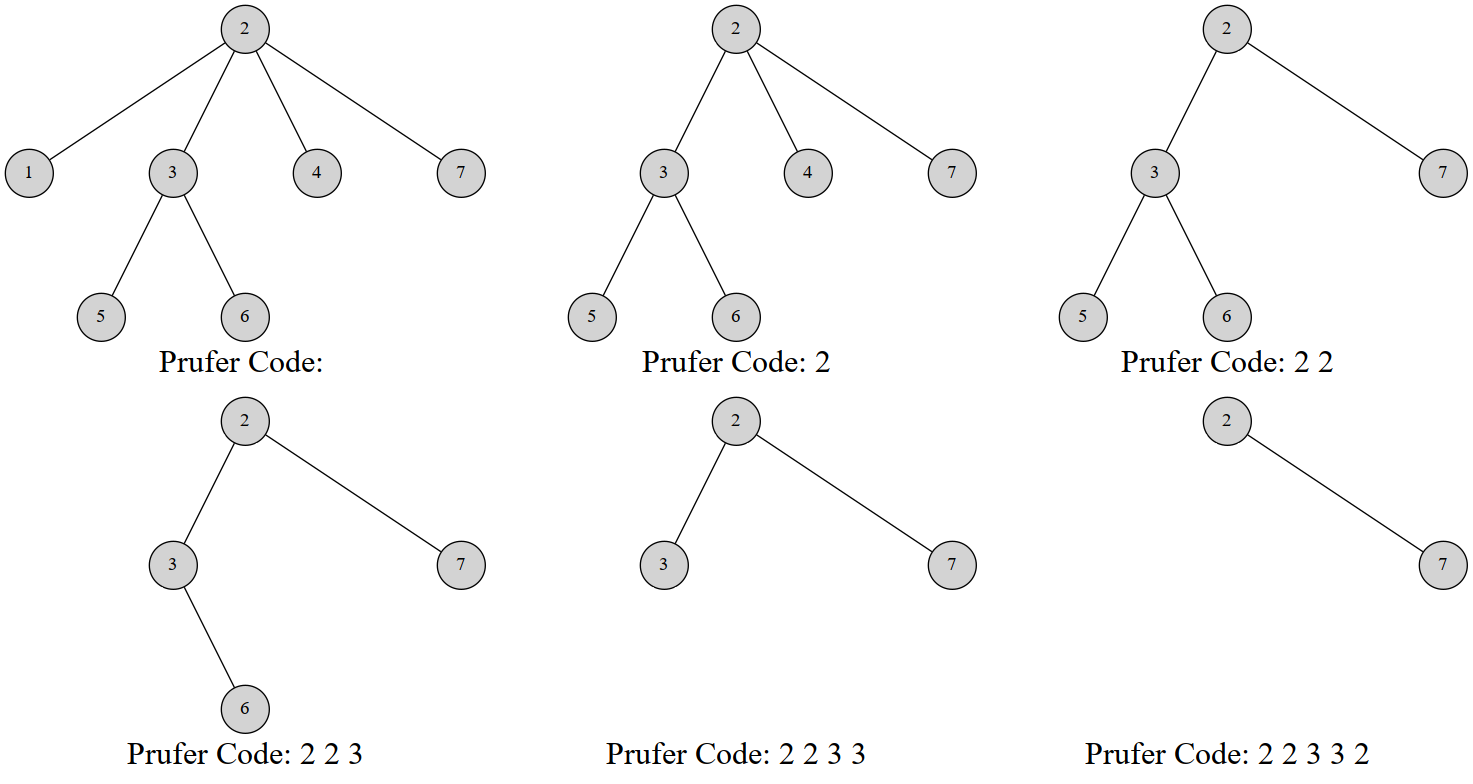# Prufer 序列

## Prufer 序列

### 引入

Prufer 序列可以将一个带标号个结点的树用中的个整数表示。你也可以把它理解为完全图的生成树与数列之间的双射。

Heinz Prufer 于 1918 年发明这个序列来证明凯莱定理。

### 对树建立 Prufer 序列

Prufer 是这样建立的：每次选择一个编号最小的叶结点并删掉它，然后在序列中记录下它连接到的那个结点。重复次后就只剩下两个结点，算法结束。

  1 2 3 4 5 6 7 8 9 10 11 12 13 14 15 16 17 18 19 20 21 22 23 24 25 26 // 代码摘自原文，结点是从 0 标号的 vector> adj; vector pruefer_code() { int n = adj.size(); set leafs; vector degree(n); vector killed(n, false); for (int i = 0; i < n; i++) { degree[i] = adj[i].size(); if (degree[i] == 1) leafs.insert(i); } vector code(n - 2); for (int i = 0; i < n - 2; i++) { int leaf = *leafs.begin(); leafs.erase(leafs.begin()); killed[leaf] = true; int v; for (int u : adj[leaf]) if (!killed[u]) v = u; code[i] = v; if (--degree[v] == 1) leafs.insert(v); } return code; } 
  1 2 3 4 5 6 7 8 9 10 11 12 13 14 15 16 17 18 19 20 21 22 23 24 25 # 结点是从 0 标号的 adj = [[]] def pruefer_code(): n = len(adj) leafs = set() degree = [] * n killed = [False] * n for i in range(1, n): degree[i] = len(adj[i]) if degree[i] == 1: leafs.intersection(i) code = [] * (n - 2) for i in range(1, n - 2): leaf = leafs leafs.pop() killed[leaf] = True for u in adj[leaf]: if killed[u] == False: v = u code[i] = v if degree[v] == 1: degree[v] = degree[v] - 1 leafs.intersection(v) return code 

### 过程### 线性构造

#### 过程

1. 删除指向的结点，并检查是否产生新的叶结点。
2. 如果产生新的叶结点，假设编号为，我们比较的大小关系。如果，那么不做其他操作；否则就立刻删除，然后检查删除后是否产生新的叶结点，重复步骤，直到未产生新节点或者新节点的编号3. 让指针自增直到遇到一个未被删除叶结点为止；

#### 性质是当前编号最小的叶结点，若删除后未产生叶结点，我们就只能去寻找下一个叶结点；若产生了叶结点• 如果，则反正往后扫描都会扫到它，于是不做操作；
• 如果，因为原本就是编号最小的，而还小，所以就是当前编号最小的叶结点，优先删除。删除继续这样的考虑直到没有更小的叶结点。

#### 实现

  1 2 3 4 5 6 7 8 9 10 11 12 13 14 15 16 17 18 19 20 21 22 23 24 25 26 27 28 29 30 31 32 33 34 35 36 37 // 从原文摘的代码，同样以 0 为起点 vector> adj; vector parent; void dfs(int v) { for (int u : adj[v]) { if (u != parent[v]) parent[u] = v, dfs(u); } } vector pruefer_code() { int n = adj.size(); parent.resize(n), parent[n - 1] = -1; dfs(n - 1); int ptr = -1; vector degree(n); for (int i = 0; i < n; i++) { degree[i] = adj[i].size(); if (degree[i] == 1 && ptr == -1) ptr = i; } vector code(n - 2); int leaf = ptr; for (int i = 0; i < n - 2; i++) { int next = parent[leaf]; code[i] = next; if (--degree[next] == 1 && next < ptr) { leaf = next; } else { ptr++; while (degree[ptr] != 1) ptr++; leaf = ptr; } } return code; } 
  1 2 3 4 5 6 7 8 9 10 11 12 13 14 15 16 17 18 19 20 21 22 23 24 25 26 27 28 29 30 31 32 33 34 35 36 # 同样以 0 为起点 adj = [[]] parent = [] * n def dfs()v: for u in adj[v]: if u != parent[v]: parent[u] = v dfs(u) def pruefer_code(): n = len(adj) parent[n - 1] = -1 dfs(n - 1) ptr = -1 degree = [] * n for i in range(0, n): degree[i] = len(adj[i]) if degree[i] == 1 and ptr == -1: ptr = i code = [] * (n - 2) leaf = ptr for i in range(0, n - 2): next = parent[leaf] code[i] = next if degree[next] == 1 and next < ptr: degree[next] = degree[next] - 1 leaf = next else: ptr = ptr + 1 while degree[ptr] != 1: ptr = ptr + 1 leaf = ptr return code 

### Prufer 序列的性质

1. 在构造完 Prufer 序列后原树中会剩下两个结点，其中一个一定是编号最大的点2. 每个结点在序列中出现的次数是其度数减。（没有出现的就是叶结点）

### 用 Prufer 序列重建树

  1 2 3 4 5 6 7 8 9 10 11 12 13 14 15 16 17 18 19 20 21 // 原文摘代码 vector> pruefer_decode(vector const& code) { int n = code.size() + 2; vector degree(n, 1); for (int i : code) degree[i]++; set leaves; for (int i = 0; i < n; i++) if (degree[i] == 1) leaves.insert(i); vector> edges; for (int v : code) { int leaf = *leaves.begin(); leaves.erase(leaves.begin()); edges.emplace_back(leaf, v); if (--degree[v] == 1) leaves.insert(v); } edges.emplace_back(*leaves.begin(), n - 1); return edges; } 

### 线性时间重建树

#### 实现

  1 2 3 4 5 6 7 8 9 10 11 12 13 14 15 16 17 18 19 20 21 22 23 24 // 原文摘代码 vector> pruefer_decode(vector const& code) { int n = code.size() + 2; vector degree(n, 1); for (int i : code) degree[i]++; int ptr = 0; while (degree[ptr] != 1) ptr++; int leaf = ptr; vector> edges; for (int v : code) { edges.emplace_back(leaf, v); if (--degree[v] == 1 && v < ptr) { leaf = v; } else { ptr++; while (degree[ptr] != 1) ptr++; leaf = ptr; } } edges.emplace_back(leaf, n - 1); return edges; } 

## 图连通方案数

Prufer 序列可能比你想得还强大。它能创造比凯莱定理更通用的公式。比如以下问题：

### 证明表示每个连通块的数量。我们对个连通块构造 Prufer 序列，然后你发现这并不是普通的 Prufer 序列。因为每个连通块的连接方法很多。不能直接淦就设啊。于是设为第个连通块的度数。由于度数之和是边数的两倍，于是。则对于给定的序列构造 Prufer 序列的方案数是